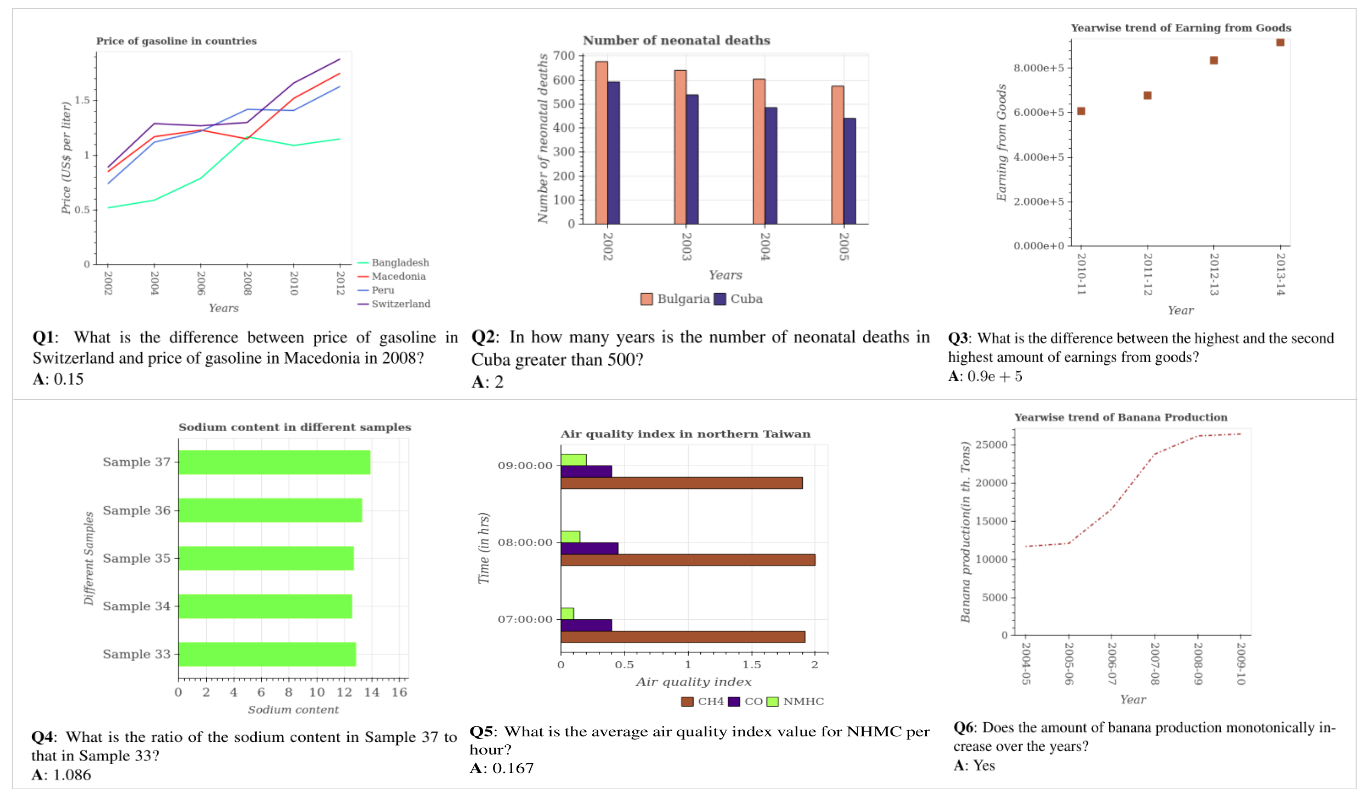Back# PlotQA

A question-answering dataset for reasoning over scientific plots.Existing synthetic datasets for reasoning over plots such as FigureQA, DVQA do not contain variability in data labels, real-valued data, or complex reasoning questions. Consequently, proposed models for these datasets do not fully address the challenge of reasoning over plots. In particular, they assume that the answer comes either from a small fixed size vocabulary or from a bounding box within the image. However, in practice this is an unrealistic assumption because many questions require reasoning and thus have real valued answers which appear neither in a small fixed size vocabulary nor in the image. In this work, we aim to bridge this gap between existing datasets and real world plots by introducing PlotQA. PlotQA is a VQA dataset with 28.9 million question-answer pairs grounded over 224,377 plots on data from real-world sources and questions based on crowd-sourced question templates.View author website